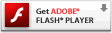# Onebyone Design

## Bayer Mosaic Filter in Pixel Bender

While just randomly browsing through wonderfl yesterday, I came across this little actionscripted bayer filter that I really liked (the one you get when you click the button labelled ‘Bayer’, that is).

Now, seeing as how I’ve been trying to learn some Pixel Bender coding lately (thanks to a little inspiration from Ralph Hauwert) I thought I’d see if I could recreate something similar with good ol’ PB. The results really aren’t too shabby and actually pretty damn fast. You can check the filter out below being applied to a Video instance (you’ll need a webcam, though). Adjust the size of the effect with the slider and decide whether or not to show color using the checkbox.

[kml_flashembed publishmethod=”static” fversion=”10.0.0″ movie=”https://blog.onebyonedesign.com/wp-content/uploads/2010/07/bayer.swf” width=”640″ height=”500″ targetclass=”flashmovie”][/kml_flashembed]

I’m sure several similar things have been done in the past, but sometimes reinventing the wheel can be a decent learning experience. If anyone would like to play around with the filter, the source is just below.

UPDATE
Got a few complaints (one in the comments below) that a null object error was being thrown when viewing the example. Just wanted to repeat that a webcam is necessary for checking it out, but I also added a quick check/error handler so that if you don’t have a webcam installed and you’re using the debug flash player, an annoying error won’t be thrown.

bayer.pbk

```

kernel NewFilter
<   namespace : "com.onebyonedesign";
vendor : "onebyonedesign.com";
version : 1;
description : "Bayer filter effect with or without color";
>
{
const float4x4 MAT = float4x4(float4(0.0, 8.0, 2.0, 10.0), float4(12.0, 4.0, 14.0, 6.0), float4(3.0, 11.0, 1.0, 9.0), float4(15.0, 7.0, 13.0, 5.0));

parameter int size
<
minValue : 1;
maxValue : 200;
defaultValue : 16;
description : "Size of the effect";
>;

parameter int useColor
<
minValue : 0;
maxValue : 1;
defaultValue : 1;
description : "use color or black and white";
>;

input image4 src;
output pixel4 dst;

void
evaluatePixel()
{

float2 c = outCoord();

// pain in the ass work around because matrix indices must be constants for flash player
int xc = int(mod(c, 4.0));
int yc = int(mod(c, 4.0));
float threshold;
if (xc == 0 && yc == 0) threshold = MAT;
if (xc == 1 && yc == 0) threshold = MAT;
if (xc == 2 && yc == 0) threshold = MAT;
if (xc == 3 && yc == 0) threshold = MAT;

if (xc == 0 && yc == 1) threshold = MAT;
if (xc == 1 && yc == 1) threshold = MAT;
if (xc == 2 && yc == 1) threshold = MAT;
if (xc == 3 && yc == 1) threshold = MAT;

if (xc == 0 && yc == 2) threshold = MAT;
if (xc == 1 && yc == 2) threshold = MAT;
if (xc == 2 && yc == 2) threshold = MAT;
if (xc == 3 && yc == 2) threshold = MAT;

if (xc == 0 && yc == 3) threshold = MAT;
if (xc == 1 && yc == 3) threshold = MAT;
if (xc == 2 && yc == 3) threshold = MAT;
if (xc == 3 && yc == 3) threshold = MAT;

pixel4 color = sampleNearest(src, outCoord());
float r = (color.r * 255.0) / float(size);
float g = (color.g * 255.0) / float(size);
float b = (color.b * 255.0) / float(size);

float avg = (r + g + b) / 3.0;
if (avg < threshold) {
if (useColor == 1) {
dst.r = color.r;
dst.g = color.g;
dst.b = color.b;
} else {
dst.r = dst.g = dst.b = 0.0;
}
} else {
dst.r = dst.g = dst.b = 1.0;
}
dst.a = 1.0;
}
}
```